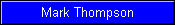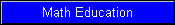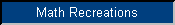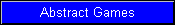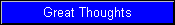During the Victorian era, Cambridge logician John Venn devised a diagram which could be used to test the validity of syllogisms, such as:1.  All mathematicians are geniuses.2.  Some chess players are mathematicians.3.  Ergo some chess players are geniuses.The categories of the statements are represented by overlapping circles.  Part of the M region is shaded to show that it has no members outside of G (statement 1), and the overlap of C and M is checked to show that there is at least one member in one of those two sections (statement 2).  Inspecting the resulting diagram shows that, since the member(s) known to exist within CM must be in CMG, there is at least one member within the regions where C and G intersect.  This shows that statement 3 is true, and so the syllogism is valid.In order to use a Venn diagram in this way, the figures representing the categories must overlap in such a way that all possible combinations are represented by distinct regions, as in the diagram shown.  Nowadays diagrams are often tailored to the particular syllogism instead of drawn in the most general form and marked up like this.  But the present puzzle is to make diagrams in which all possible combinations of overlaps are present, using not circles but another type of shape familiar in recreational math, called polyominos.A polyomino is a figure formed by joining congruent squares along their edges.  For example, a domino is a polyomino of two squares, and polyominos of other orders are named by analogy (see right).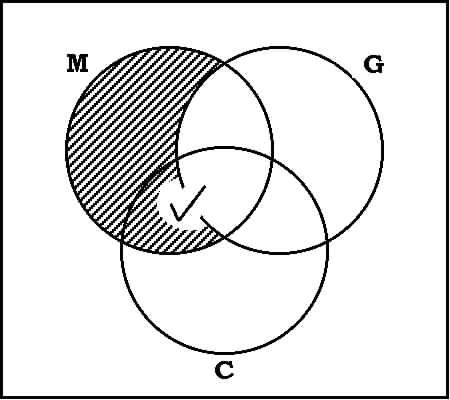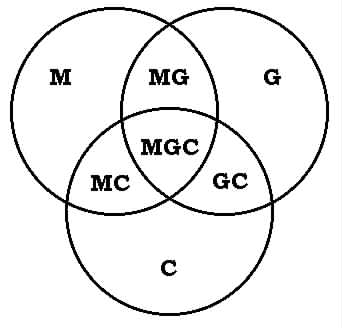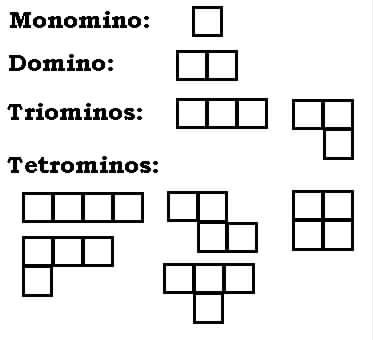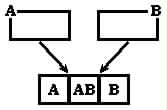Is it possible to form a Venn-like diagram out of congruent polyominos -- that is, to position a number of polyominos in such a way that every possible combination of overlaps is represented by a unit square?The simplest case is formed with only two dominos, A and B, which overlap to produce all three possible combinations.I call this a 2nd-order Venn Polyomino diagram.  To produce a 3rd-order Venn Polyomino diagram, one must turn to the tetrominos.  For example, the diagram at right shows how three L-shaped tetrominos can combine to form all seven possible combinations.Can you form 3rd-order Venn Polyomino diagrams using other tetrominos?  Two of the remaining four tetrominos have solutions.Also, can you find a 4th-order Venn Polyomino diagram, using four congruent octominos?  One example is shown at right, using a rather uninteresting 2x4 octomino.  I also know a more interesting solution. I suspect that many solutions are possible, but they're rather hard to find.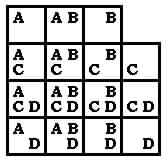Questions, corrections, comments:  Send me e-mail at  markthom@flash.net[Math Education] [Math Recreations] [Abstract Games] [Great Thoughts]